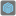• # Help: different results @web vs @pycharm

## Question related to missionUnfair Dice

I got an error message when ran check on the web page for my scripts. It says: Your result: [] There are dice that can beat this one. Fail: checkio([3,3,3])

However, when I run the same script on pycharm 2018.1 community edition, it can pass and generate an output as [1, 4, 4]. My script is below. Can someone from checkio help check what's wrong? Thanks.

```def divide(number, count, output=[], array = []):
if count == 1:
array.append(number)
array.sort()
if array not in output:
output.append(array)
yield array
else:
for i in range(1,number):
array_2 = array.copy()
array_2.append(i)
for i in divide(number - i, count - 1, output, array_2.copy()):
yield i

def win(die_1, die_2):
probabilities_1 = {i: die_1.count(i) / len(die_1) for i in set(die_1)}
probabilities_2 = {i: die_2.count(i) / len(die_2) for i in set(die_2)}
win_chances = 0
lose_chances = 0
for i in probabilities_1:
for j in probabilities_2:
if i > j:
win_chances += probabilities_1[i] * probabilities_2[j]
elif i < j:
lose_chances += probabilities_1[i] * probabilities_2[j]
else:
pass # no need to take care of the equal case as it will turn out to be win or lose.

if float("{0:.2f}".format(win_chances)) > float("{0:.2f}".format(lose_chances)):
return True

def winning_die(enemy_die):
for i in divide(sum(enemy_die), len(enemy_die)):
if win(i, enemy_die):
return i

return []
```16